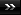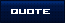Thread: Cracking the Code View Single Post11-12-2008, 05:34 AM   #61
herbivore
Avalon Senior Member

Join Date: Oct 2008
Location: va, us
Posts: 97Re: Cracking the Code

Quote:
 Originally Posted by GregorArturoI came across something inteseting while trying to ratify the concept if you can turn infinties into quantum numbers, specifically divisors of 9. Anyways, its seem that all factors that have a multiple of 3 as a numerator and a non-multiple of 3 as the denominator, then you can multiply the numerator and denomator together to get the quantum number. Just another example of why 3,6,& 9 are so bad @\$\$ haha. For example: Fraction Shortcut: 6/5 = 6*5 = 30 = 3+0 = 3 Traditional Way: 6/5 = 1.2 = 1+2 = 3 I tested it with a bunch of numbers and it seems pretty solid as long as the fraction doesn't produce an irrational number. The irrational numbers was what I was trying to define. However, let's take a look at the multiplication pattern of 7 (factors of 7): Second number after comma is the quantum number 7*1 = 7,7 7*2 = 14,5 7*3 = 21,3 7*4 = 28,1 7*5 = 35,8 7*6 = 42,6 7*7 = 49,4 7*8 = 56,2 7*9 = 63,9 Now think of this: 3/7=3*7=21=2+1=3. 6/7=6*7=42=4+2=6. Let's now look at 3. 3*1 = 3,3 3*2 = 6,6 3*3 = 9,9 3*4 = 12,3 3*5 = 15,6 3*6 = 18,9 3*7 = 21,3 3*8 = 24,6 3*9 = 27,9 Now you have nine thirds (aka nine one-thirds) that make up the number 3, or 9/3 (trying to make this sound simple). Apply the table to those nine-thirds, with the same notion of 7 in mind: 1/3=1*3=3 5/3=5*3=15=1+5=6 7/3=7*3=21=1+2=3 So this means we can now solve the quantum number for irrational numbers (aka infinities) as long as it can be written as a fraction! Hell ya! Also, this made me think of my ultimate math question which I can't deduce with any math: Point nine repeating... aka .999999999999999999999999999999999999999999999 Now mathematicans will say that equals 9/9 or 1 but think about it; even if it does repeat on forever it's not 1! That's a mind *uck I tell ya.
the repeating 9s HAVE to have some relation to 9s significance. an infinite 9s! whichever numbers have the .99999... have a place in all of this.